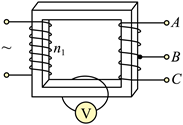$\text{A.}$ $n_1$ 为 1100 匝, $U_{\mathrm{m}}$ 为 $220 \mathrm{~V}$ $\text{B.}$ $B C$ 间线圈匝数为 120 匝, 流过 $R$ 的电流为 $1.4 \mathrm{~A}$ $\text{C.}$ 若将 $R$ 接在 $A B$ 两端, $R$ 两端的电压为 $18 \mathrm{~V}$, 频率为 $100 \mathrm{~Hz}$ $\text{D.}$ 若将 $R$ 接在 $A C$ 两端, 流过 $R$ 的电流为 $2.5 \mathrm{~A}$, 周期为 $0.02 \mathrm{~s}$

D

#### 解析：

A. 变压器的输入电压为 $220 \mathrm{~V}$, 原线圈的交流电的电压与时间成余弦函数 关系, 故输入交流电压的最大值为 $220 \sqrt{2} \mathrm{~V}$, 根据理想变压器原线圈与单匝线圈的匝数比 为 $\frac{n_1}{1}=\frac{220 \mathrm{~V}}{0.1 \mathrm{~V}}$.

B. 根据图像可知, 当原线圈输入 $220 \mathrm{~V}$ 时, $B C$ 间的电压应该为 $12 \mathrm{~V}$, 故 $B C$ 间的线圈匝数 关系有
$$\frac{n_{B C}}{1}=\frac{12 \mathrm{~V}}{0.1 \mathrm{~V}}=120$$

$B C$ 间的线圈匝数为 120 匝, 流过 $R$ 的电流为 $I_{B C}=\frac{P}{U_{B C}}=\frac{12 \mathrm{~W}}{12 \mathrm{~V}}=1 \mathrm{~A}$ B 错误;
C. 若将 $R$ 接在 $A B$ 端，根据图像可知，当原线圈输入 $220 \mathrm{~V}$ 时， $A B$ 间的电压应该为 $18 \mathrm{~V}$ 。 根据交流电原线圈电压的表达式可知, 交流电的角速度为 $100 \pi$, 故交流电的频率为 $f=\frac{1}{T}=\frac{\omega}{2 \pi}=50 \mathrm{~Hz} \mathrm{C}$ 错误；

D. 若将 $R$ 接在 $A C$ 端, 根据图像可知, 当原线圈输入 $220 \mathrm{~V}$ 时, $A C$ 间的电压应该为 $30 \mathrm{~V}$, 根据欧姆定律可知, 流过电阻 $R$ 的电流为 $I_{A C}=\frac{U_{A C}}{R}=\frac{30}{12} \mathrm{~A}=2.5 \mathrm{~A}$ 交流电的周期为
$T=\frac{1}{f}=\frac{2 \pi}{\omega}=0.02 \mathrm{~s}$ D 正确。选 D。